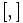# Nilpotency class

(Redirected from Nilpotency class of a group)

This article defines an arithmetic function on a restricted class of groups, namely: nilpotent groups

## Definition

### Equivalent definitions in tabular format

The notion of nilpotency class (also called nilpotence class) makes sense for any nilpotent group and is a nonnegative integer dependent on the group.

No. Shorthand The nilpotency class is defined as ... The nilpotency class of a nilpotent group$G$ is...
1 upper central series the length of the upper central series the smallest nonnegative integer$c$ such that$Z^c(G) = G$. Here, we define$Z^c(G)$ inductively as follows:$Z^c(G)$ is the inverse image of the center$Z(G/Z^{c-1}(G))$ under the natural quotient map from$G$ to$G/Z^{c-1}(G)$, and$Z^0(G)$ is the trivial subgroup.

2 lower central series the length of the lower central series the smallest nonnegative integer$c$ such that$[[[..[G,G],G],G],...G]$ is trivial where$G$ is repeated$c + 1$ times. Here,$[,]$ denotes the commutator of two subgroups. In other words, the lower central series of$G$ reaches the identity in finitely many steps.
3 central series the minimum possible length of a central series the smallest nonnegative integer$c$ and a chain of subgroups:$G = H_1 \ge H_2 \ge \dots \ge H_{c+1} = \{ e \}$ such that each$H_i$ is a normal subgroup of$G$ and$H_i/H_{i+1}$ is in the center of$G/H_{i+1}$. In other words, there exists a central series for$G$ of length$c$.
4 diagonal subnormal in square the subnormal depth of the diagonal subgroup in the square of the group the subnormal depth of the subgroup$\{ (g,g) : g \in G \}$ in the square$G \times G$ with subnormal depth$c$.
5 iterated left-normed commutators trivial the smallest length such that any iterated left-normed commutator of length more than that becomes trivial the smallest length$c$ such that any commutator of the form$[[\dots[x_1,x_2],x_3],\dots,x_{c+1}]$ takes value the identity element, where the$x_i$ are (possibly repeated) elements of$G$.
6 iterated commutators of any form trivial the smallest length such that any iterated commutator (with any kind of parenthesization of terms) of length more than that becomes trivial the smallest length$c$ such that any iterated commutator that involves at least$c$ commutator operations (so$c+1$ original inputs) takes value the identity element. [SHOW MORE]
7 iterated left-normed commutators trivial (generating set version) (pick a generating set for the group) the smallest finite length such that any iterated commutator (with any kind of parenthesization of terms) of length more than that becomes trivial (pick a generating set$S$ for$G$) the smallest finite length$c$ such that any iterated commutator that involves at least$c$ commutator operations (so$c+1$ original inputs) and where the inputs are from$S$, always takes value the identity element. [SHOW MORE]

### Equivalence of definitions

For full proof, refer: Equivalence of definitions of nilpotency class

### Terminology

When we say that a group has nilpotency class$c$, we usually mean that the nilpotency class of the group is at most equal to$c$. If we want to say that the class is exactly$c$, this is stated explicitly.

## Particular cases

Value of$c$ Name for groups of nilpotency class at most$c$
0 trivial group only
1 abelian groups (note that nontrivial abelian groups have class exactly 1)
2 group of nilpotency class two. The non-abelian ones among these have class exactly 2
3 group of nilpotency class three

## Example

Any group of prime power order is nilpotent. Further information: prime power order implies nilpotent

A group of order$p^n$, with$p$ prime, can have any nilpotency class between 1 and$n - 1$ if$n \ge 2$. For more information of the number of p-groups of various nilpotency class values for various primes, refer nilpotency class distribution of p-groups.

## Relation with other arithmetic functions

### Relation with derived length

Further information: Nilpotency class versus derived length

Any nilpotent group is solvable, and there are numerical relations between the nilpotency class and derived length: